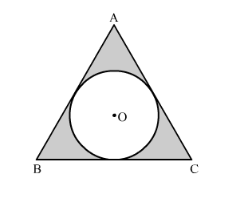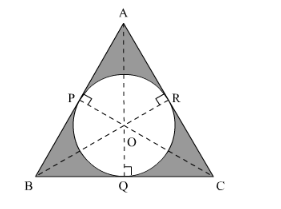# A circle is inscribed in an equilateral triangle ABC is side 12 cm,Question:

A circle is inscribed in an equilateral triangle ABC is side 12 cm, touching its sides (the following figure). Find the radius of the inscribed circle and the area of the shaded part.Solution:

We have to find the area of the shaded portion. We have $\triangle \mathrm{ABC}$ which is an equilateral triangle and $\mathrm{AB}=12 \mathrm{~cm}$.We have O as the incentre and OP, OQ and OR are equal.

So,

$a r(\Delta \mathrm{ABC})=a r(\Delta \mathrm{OAB})+a r(\Delta \mathrm{OBC})+a r(\Delta \mathrm{OCA})$

Thus,

$\frac{\sqrt{3}}{4}(12)^{2}=3\left(\frac{1}{2}(12)(r)\right)$

$r=\frac{36 \sqrt{3}}{18} \mathrm{~cm}$

$=2 \sqrt{3} \mathrm{~cm}$

So area of the shaded region,

$=\operatorname{ar}(\triangle \mathrm{ABC})-$ Area of the circle

$=\frac{\sqrt{3}}{4}(12)^{2}-\frac{22}{7}(2 \sqrt{3})^{2}$

$=(62.35-37.71) \mathrm{cm}^{2}$

$=24.64 \mathrm{~cm}^{2}$